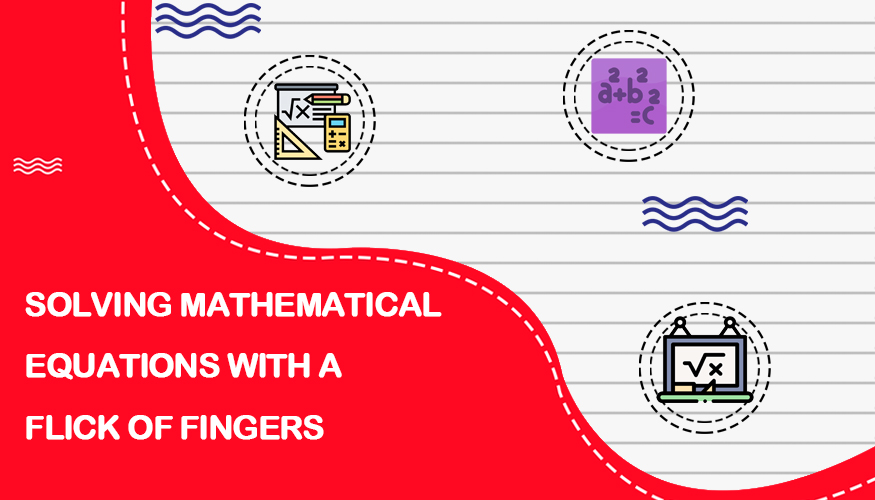### Eco-Friendly Washing Machine: A Workout and Laundr# Hello worldMany students find maths as a difficult subject because it has a lot of difficult calculations. Long calculations takes a lot time to be solved. To overcome this and save time shortcuts are used in maths. Shortcuts are simple way or trick through which bigger problems get solved easily.

Math can be difficult for many students. The Common Core State Standards in Mathematics (CCSSM) define what students should understand and be able to do in their study of mathematics. The aim of these standards is that all students should learn important mathematical concepts and processes with understanding. They recognize that while learning specific content in mathematics is important, developing the skills of thinking and reasoning are equally important. Unfortunately, the grade-specific standards do not define the intervention methods or materials necessary to support students who are well below or well above grade-level expectations. Teachers must learn interventions skills in order to help these students.

Interventions have become an important way for teachers to ensure that all students succeed in today?s high stakes testing environment. Easy and effective math tips can help teachers identify who may benefit from intervention and address the needs of those students. Listed below are four categories of math shortcut strategies teachers can use to make math easier for their students.

The order of the numbers in an addition problem does not affect the answer.

When zero is added to the original number, the answer is the original number.

When 1 is added to the original number, the answer is the next larger number.

### 2. Subtraction

Teachers can teach students these shortcuts to help with basic subtraction:

When zero is subtracted from the original number, the answer is the original number.

When 1 is subtracted from the original number, the answer is the next smaller number.

When the original number has the same number subtracted from it, the answer is zero

### 3. Multiplication

These multiplication shortcuts can be taught to the students:

When a number is multiplied by zero, the answer is zero.

When a number is multiplied by 1, the answer is the original number.

When a number is multiplied by 2, the answer is equal to the number being added to itself.

The order of the numbers in a multiplication problem does not affect the answer.

### 4. Division

Teachers can teach students these division shortcuts:

When zero is divided by any number, the answer is zero.

When a number is divided by 1, the answer is the original number.

When a number is divided by itself, the answer is 1.

Children?s Use of Arithmetic: The Role of Preference in Strategy Choice Children?s understanding of the relations between addition and subtraction is considered integral for later mathematical skills . Part of the research agenda needs to understand and account for the large individual differences in children?s conceptual knowledge . While many researchers have focussed on factors such as general mathematics skills or working memory to account for individual differences in understanding the relationship between addition and subtraction, one overlooked factor may be students? attitudes and beliefs towards mathematics. Attitudes are positive or negative evaluations (e.g., ?I like addition?) and beliefs are ?thoughts? based on experience (e.g., ?division is difficult?) and both are considered to be integral in determining success in mathematics . These attitudes and beliefs could be integral in the conceptual knowledge that children use and understand when making problem solving strategy choices.

Researchers have often considered children?s use of the inversion shortcut strategy on inversion problems of the form a + b - b as an indicator of understanding of the inverse relation between the two operations . The inversion shortcut involves stating that the answer is??without performing any calculations as the two??terms cancel each other out. Children?s understanding of the relationship between addition and subtraction can also be assessed via children?s use of the associativity shortcut on problems of the form a + b - c , which were originally included in studies of children?s inversion as control problems . When children understand that addition and subtraction are associative, they can use that information to simplify and speed up problem solving by first subtracting and then adding (e.g., 3 +29+27 would be solved by calculating 29-27 and then adding 3) . Both inversion and associativity three-term problems are novel and therefore children must implement spontaneous problem solving strategies . Researchers have taken advantage of this novelty to gain an understanding of children?s knowledge of the relations between addition and subtraction by utilizing children?s use of conceptually-based shortcuts as an indicator of conceptual understanding because understanding the inverse or associative relations between addition and subtraction is required to implement the inversion and associativity shortcuts .

Given the importance of understanding the relations between addition and subtraction, some studies have gone beyond assessing children?s spontaneous use or evaluation of the inversion shortcut by trying to promote conceptually-based shortcut use focussing exclusively on inversion problems. In these studies, 8-year-old children?s conceptual understanding of the inverse relation between addition and subtraction, as assessed via shortcut use increased after either 2 or 3 training sessions with concrete objects [10, 11] or after repeated weekly exposure to inversion problems.

A possible alternative to the longer interventions used in previous studies comes from a study with Grade 2, 3, and 4 students by Robinson and Dub